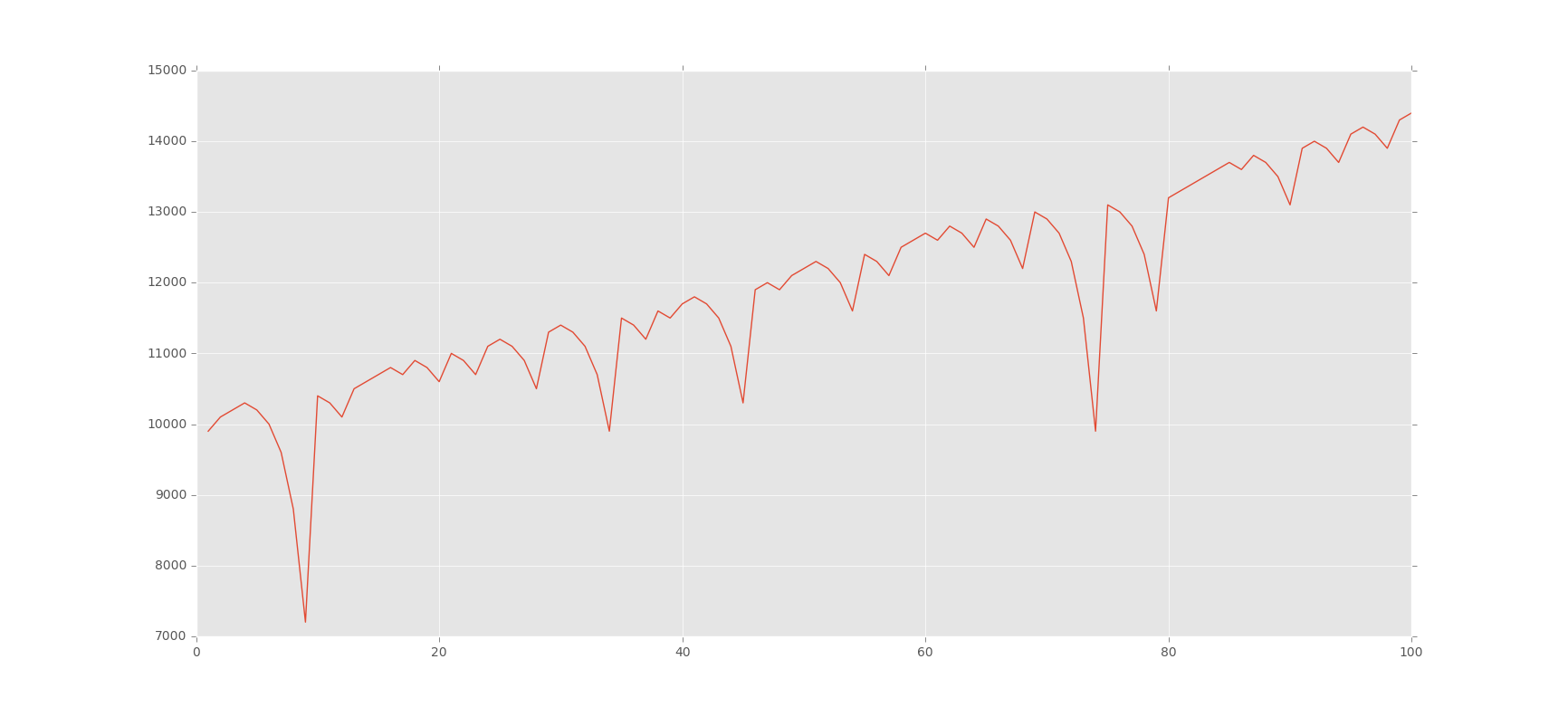## Martingale Strategy

One of the most common bright ideas people get is to double up on losses. Thus, if you lose, you just simply double the previous wager amount. You continue this until a win.

If you have unlimited money, this strategy works, but you don't, so it doesn't. All this strategy does is accelerates the process. Again, each time you wager, you face whatever the odds are. You cannot avoid them. Regardless, we're going to illustrate it!

```import random
import matplotlib
import matplotlib.pyplot as plt
#
import time

def rollDice():
roll = random.randint(1,100)

if roll == 100:
return False
elif roll <= 50:
return False
elif 100 > roll >= 50:
return True

def doubler_bettor(funds,initial_wager,wager_count):
value = funds
wager = initial_wager
wX = []
vY = []
currentWager = 1

# since we'll be betting based on previous bet outcome #
previousWager = 'win'

# since we'll be doubling #
previousWagerAmount = initial_wager

while currentWager <= wager_count:
if previousWager == 'win':
print 'we won the last wager, yay!'
if rollDice():
value += wager
print value
wX.append(currentWager)
vY.append(value)
else:
value -= wager
previousWager = 'loss'
print value
previousWagerAmount = wager
wX.append(currentWager)
vY.append(value)
if value < 0:
print 'went broke after',currentWager,'bets'
currentWager += 10000000000000000
elif previousWager == 'loss':
print 'we lost the last one, so we will be super smart & double up!'
if rollDice():
wager = previousWagerAmount * 2
print 'we won',wager
value += wager
print value
wager = initial_wager
previousWager = 'win'
wX.append(currentWager)
vY.append(value)
else:
wager = previousWagerAmount * 2
print 'we lost',wager
value -= wager
if value < 0:
print 'went broke after',currentWager,'bets'
currentWager += 10000000000000000
print value
previousWager = 'loss'
previousWagerAmount = wager
wX.append(currentWager)
vY.append(value)
if value < 0:
print 'went broke after',currentWager,'bets'
currentWager += 10000000000000000

currentWager += 1

print value
plt.plot(wX,vY)

doubler_bettor(10000,100,100)
plt.show()
time.sleep(555)

'''
Simple bettor, betting the same amount each time.
'''
def simple_bettor(funds,initial_wager,wager_count):
value = funds
wager = initial_wager
wX = []
vY = []
currentWager = 1
while currentWager <= wager_count:
if rollDice():
value += wager
wX.append(currentWager)
vY.append(value)
else:
value -= wager
wX.append(currentWager)
vY.append(value)
currentWager += 1
plt.plot(wX,vY)
x = 0

while x < 100:
simple_bettor(10000,100,1000)
x += 1

plt.ylabel('Account Value')
plt.xlabel('Wager Count')
plt.show()

```The next tutorial:• Monte Carlo Introduction

• Monte Carlo dice Function

• Creating a simple Bettor

• Plotting Results with Matpltolib

• Martingale Strategy
• Bettor Statistics

• More comparison

• Graphing Monte Carlo

• Fixing Debt Issues

• Analyzing Monte Carlo results

• Using Monte Carlo to find Best multiple

• Checking betting results

• D'Alembert Strategy

• 50/50 Odds

• Analysis of D'Alembert

• Comparing Profitability

• Finding best D'Alembert Multiple

• Two dimensional charting monte carlo

• Monte Carlo Simulation and Python

• Labouchere System for Gambling Tested